# Adaptive and Feature-Preserving Bilateral Filters for Three-Dimensional Models

Adaptive and Feature-Preserving Bilateral Filters for Three-Dimensional Models
Department of Information Science and Technology, Dalian Maritime University, Dalian 116085, China

Corresponding Author Email:
bojiang@dlmu.edu.cn
Page:
157-168
|
DOI:
https://doi.org/10.18280/ts.370202
18 November 2019
|
Accepted:
23 February 2020
|
Published:
30 April 2020
| Citation

OPEN ACCESS

Abstract:

Bilateral filtering is a well-known tool to denoise or smooth one-dimensional (1D) signals, two-dimensional (2D) images, and three-dimensional (3D) models. The bilateral weights help preserve the edges or features more effectively than unilateral weights. However, it is immensely difficult to configure the scale parameters of the convolutional kernel functions. To overcome the difficulty, this paper proposes adaptive, feature-preserving bilateral filters for 3D models by introducing automatic, adaptive scale parameters. Firstly, the feature scale was defined on 3D models, bridging up the gap between feature scale and scale parameter of the Gaussian functions. Next, the scale descriptor was proposed to adaptively configure the scale parameter for each face of the target 3D model, changing the traditional approach of adopting the same scale parameter for all faces. On this basis, a feature-preserving local filter was designed by introducing the adaptive scale parameters to the iterative local scheme, and a modified global filter, which is robust to irregular sampling during denoising, was designed based on the adaptive scale parameters. The excellence of our filters was proved through experiments on multiple synthetic and real-world noisy models, in comparison to the state-of-the-art filters. The research results lay a solid basis for feature preservation and noise removal of 3D models.

Keywords:

bilateral filtering, mesh denoising, scale parameters, feature preservation

1. Introduction

Digital scanners have been widely adopted to collect three-dimensional (3D) points on curved surfaces. During the data collection, various noises are inevitably introduced. As a result, mesh denoising becomes a research hotspot in geometric processing. Mesh denoising aims to remove noises without sacrificing the features that are useful in fields like rendering, scientific analysis, and medical visualization.

Bilateral filtering is a basic yet important tool for denoising 3D mesh models. In traditional bilateral filters, however, it is difficult to configure the scale parameters of convolutional functions. The difficulty arises from the local average nature of bilateral filtering. Improper scale parameters will either remove the model features or fail to eliminate the noises. Thus, the key to mesh denoising is to remove the noises while preserving or recovering model features. In this paper, an adaptive method is proposed to configure the scale parameters, and used to design two adaptive, feature-preserving bilateral filters.

So far, many bilateral filtering methods have been designed for mesh denoising. The most common ones are local methods [1-3]. In local methods, the model is denoised iteratively by weighting and averaging local geometric elements. The number of iterations must be selected manually. If it is too small, the noises cannot be fully removed; if it is too large, the model features will get lost along with the noises. To solve the problems in local methods, global methods have been developed [4, 5], which maintain the overall structure of model by considering the global features. However, the global methods cannot preserve the local details or reduce heavy noises.

Recent methods tend to add one or more pre- or post-processing steps to local or global methods, such as pre-filtering and feature detection. Despite their good results, these multi-step methods consume lots of time, calling for frequent parameter adjustments and manual interventions. Deep learning (DL) [6, 7] have also been successfully applied to mesh denoising. However, DL methods require prolonged trainings, and their generalization ability varies with the networks. Only models included in the training set could be preserved well by DL methods. Hence, it is an urgent problem to find a simple way to denoise mesh models.

Guidance filtering  provides a way to improve bilateral filtering: the guidance signal is extracted from the original model, and used to modify the bilateral weights for feature preservation. There are also strategies  that analyze the scale space of Gaussian functions. Drawing on these works, this paper attempts to modify bilateral filtering on mesh models from the perspective of scale space, and preserve the features of different scales based on the features of Gaussian scale space.

In this paper, two adaptive, feature-preserving bilateral filters are developed for 3D mesh models, based on the automatic and adaptive settings of the scale parameters. Firstly, the scale of 3D model features was defined, bridging the gap between feature scales and scale parameters of bilateral filtering. Next, the scale descriptor was introduced to enable the adaptive setting of scale parameters. The adaptive scale parameters were incorporated into local and global methods, improving their feature preserving ability. The primary contributions of this paper are as follows:

(1) The traditional bilateral filters were improved through automatic and adaptive configuration of scale parameters, solving the scale-sensitive problem.

(2) Feature scales and scale descriptors were defined for the construction of adaptive scale parameters.

(3) Adaptative local and global bilateral filters were designed, both of which are feature-preserving, and robust to extremely noisy models and irregular mesh sampling.

2. Literature Review

The existing mesh denoising methods fall into four categories: local methods, global methods, multi-step methods, and DL methods.

2.1 Local methods

Local methods are classical algorithms in the field of denoising. In essence, local methods remove noises based on the weighted average of triangular neighborhoods. The effectiveness of local methods is commonly improved by optimizing the convolution kernel.

To improve the bilateral filtering of images, Fleishman et al.  optimized the kernel function based on the differences of vertex positions and face normal. Zheng et al.  achieved more detailed results by improving the kernel, in the light of centroid distance and normal difference. Liu et al.  improved the kernel according to the normal difference of geodesic distances. To prevent the diffusion of sharp edge, Yadav et al.  optimized the Gaussian kernel function by the Tukey’s bi-weight function. Zhang et al.  extracted the guidance signal from the original model, and replaced the original signal with the extracted signal, thereby improving the kernel function.

Centin and Signoroni  updated the normal direction based on the local and global curvature changes of the model, and thus maintained and enhanced the model features. Wang et al.  extended the method of Zheng et al.  into rolling guidance filtering , in which the original normal direction serves as the guide in each iteration to preserve the features of the original model. Through feature detection, Liu et al.  identified feature surfaces and non-feature surfaces, and proposed neighborhood selection metrics to enhance features; their method achieved better results than guided filtering.

The bilateral filtering effect can also be improved by selecting triangular neighborhoods for the mesh model. For example, Sun et al.  selected neighborhoods based on the normal angles of triangle patches and neighborhood patches. Li et al.  preserved sharp features through edge-based neighborhood processing. Hurtado et al.  considered gradient, point, and normal information to select and process neighborhoods. Overall, local methods can recover model features iteratively. But the setting of the number of iterations remains a difficulty.

2.2 Global methods

In recent years, global methods have attracted much interest from the academia. For instance, Zheng et al.  designed an isotropic denoising method based on bilateral weighted Laplacian operator. However, the Laplacian operation cannot distinguish weak features from noises, nor effectively handle models with heavy noises. To overcome the defects, He and Schaefer  proposed a minimal mesh denoising algorithm based on edge operators, while Zhang et al.  created the total variation (TV) normal filter. But the two methods, especially the former, both suffer from undesired staircase effect in gently curved regions. Later, Zhao et al.  optimized the nonconvex solution process, and proposed an upgraded alternating strategy to solve minimal mesh denoising. Inspired by the theory of compressed perception, Wang et al.  recovered model features from the residuals of Laplacian factorization.

Recently, the second-order normalization has been widely adopted in global methods. For example, Liu et al.  combined the second-order normalization with normal fidelity terms. On this basis, Zhong et al.  preserved the details of the original model well, in view of the overall variational differences and the fidelity of the normal. To sum up, global methods are good at retaining the overall structure of the model, but poor in maintaining the details on local geometry.

2.3 Multi-step methods

Feature detection is often conducted first to preserve the model features in the course of denoising, which gives rise to multi-step methods. One of the most popular ways to identify noises and features of mesh models is feature detection based on tensor voting. Wei et al.  processed noises and features differently by voting on the types of points based on multi-scale normal tensor. Yadav et al.  introduced the concept of local binary neighborhood to realize binary optimization of the tensor equation, and successfully maintained the model features.

The existing multi-step methods have been optimized by various methods, namely, global filtering [24-26], local filtering , median filtering , feature detection [24, 29, 30], and neighborhood searching . Typical multi-step methods [24, 29, 30] added feature detection to the filtering process or the point updating process. Wei et al.  improved the denoising effect by combining the merits of point normal field and surface normal field, which are consistent in local areas. Lu et al.  integrated the global method with L1-median filtering to remove noise. In summary, multi-step methods offer meticulous means to preserve model features. However, the feature preservation comes at the cost of high computing complexity and frequent parameter adjustments.

2.4 DL methods

DL methods have also been successfully applied to mesh denoising.

For instance, Wang et al.  proposed a mesh filter with one-step learning: the filter learns cascaded non-linear regression functions from a set of noisy meshes and their ground-truth counterparts.

Wang et al.  designed a two-step learning mechanism for the mesh filter: in the first step, the neural network learns the mapping function from a set of noisy models and their ground-truth counterparts; in the second step, the ground-truth of filtered models are learned to recover the geometric loss in the first step.

Despite their good performance, DL methods face several problems in feature preservation: the training is very time-consuming, and the training results are greatly affected by the training set (i.e. the features not included in the training set cannot be recovered).

3. Bilateral Filter and Scale Parameters

3.1 Original bilateral filter on two-dimensional (2D) images

Tomasi and Manduchi  were to first to propose bilateral filtering for image processing. The filtering was performed based on the spatial positions and pixel values of the target image. For the target image I, the bilateral filtering at the pixel p=(x, y) can be defined as:

$I(\widehat{p})=\frac{\sum\nolimits_{q\in N(p)}{\omega _{c}^{I}}(||p-q||)\omega _{s}^{I}(||I(p)-I(q)||)I(q)}{\sum\nolimits_{q\in N(p)}{\omega _{c}^{I}}(||p-q||)\omega _{s}^{I}(||I(p)-I(q)||)}$  (1)

where, N(p) is the neighborhood of pixel p; $\omega_{c}^{I}(x)$ and $\omega_{S}^{I}(x)$ are Gaussian functions:

\left\{ \begin{align} & \omega _{c}^{I}(||p-q||)=\exp (-\frac{{{(||p-q||)}^{2}}}{{{(\sigma _{c}^{I})}^{2}}}) \\ & \omega _{s}^{I}(||I(p)-I(q)||)=\exp (-\frac{{{(||I(p)-I(q)||)}^{2}}}{{{(\sigma _{s}^{I})}^{2}}}) \\\end{align} \right.  (2)

where, $\sigma_{C}^{I}$ and $\sigma_{s}^{I}$ are the scale parameters of the Gaussian functions on spatial positions and pixel values; the multiplication operators are all numerical multiplications.

3.2 Bilateral filter on 3D mesh models

Most mesh denoising methods target triangular mesh models. Let fi(i=1, 2, ..., M) be the face i of the target 3D model, and M be the number of faces on the model. Then, the normal ni of face fi can be defined on the centroid ci of the face. Based on face normals, the classical filtering algorithm proposed Zhang et al.  converts the original bilateral filter on 2D images into a bilateral filter for 3D mesh models:

$n_{i}^{t+1}=\frac{\sum\nolimits_{j\in N(i)}{{{\omega }_{c}}(||{{c}_{i}}-{{c}_{j}}||){{\omega }_{s}}(||n_{i}^{t}-n_{j}^{t}||){{\xi }_{ij}}n_{j}^{t}}}{\sum\nolimits_{j\in N(i)}{{{\omega }_{c}}(||{{c}_{i}}-{{c}_{j}}||){{\omega }_{s}}(||n_{i}^{t}-n_{j}^{t}||){{\xi }_{ij}}}}$  (3)

where, $n_{i}^{t}$ is face normal i obtained in the t-th iteration; ξij is a parameter related to sampling rate; ωc(x) and ωs(x) are the Gaussian kernel functions based on spatial distance and normal difference, respectively:

$\left\{ \begin{matrix} {{\omega }_{c}}(||{{c}_{i}}-{{c}_{j}}||)=\exp (-\frac{||{{c}_{i}}-{{c}_{j}}|{{|}^{2}}}{2\sigma _{c}^{2}}) \\ {{\omega }_{s}}(||n_{i}^{t}-n_{j}^{t}||)=\exp (-\frac{||n_{i}^{t}-n_{j}^{t}|{{|}^{2}}}{2\sigma _{s}^{2}}) \\\end{matrix} \right.$  (4)

where, σc and σs are the scale parameters of the corresponding Gaussian kernel functions.

3.3 Scale-based feature analysis

The above analysis demonstrates the importance of scale parameters to bilateral filters. Any change to the scale parameters will affect how an element (pixel or vertex) is influenced by its neighbors. Here, the scale parameter in the spatial domain is named the spatial scale parameter, and that in the value domain (pixel value or face normal value) is named as the value scale parameter. The former is more stable than the latter in most problems. Thus, bilateral filtering tasks usually focus on the configuration of the value scale parameter. In this paper, the spatial scale parameter is empirically set as the mean distance between the centroid of each face and the centroids of its neighbors. Therefore, the value scale parameter is referred to as the scale parameter for short.

The scale parameter directly bears on the filtered values in different tasks. Thus, this paper proposes the concept of multi-scale features on the mesh model. The filtering process on the 3D model with Gaussian function ωs(x) of variance σs was denoted as Ls(n), i.e. the filtering process in equation (3), where $\omega_{s}=\exp \left(-\frac{x^{2}}{2 \sigma_{S}^{2}}\right)$, $L_{S}$ is the filtering result on the scale σs, and n=(n1,n2,…,nM) is the face normals of the entire model. The authors considered this feature on scale σs, such that the corresponding structures disappear when the Gaussian function of variance σs is applied to the model.

Figure 1 shows the results of bilateral filtering on a one-dimensional (1D) signal, a 2D image, and a 3D model at different values of the scale parameter. Obviously, the features on different scales were gradually removed, with the growth in the scale parameter. As shown in the sub-graphs, features on different scales were removed at different scale parameters. For the bunny model, the muscle textures belong to scale σs=0.3, the eye belongs to scale σs=0.5, and the paw belongs to scale σs=1.

## 1.jpg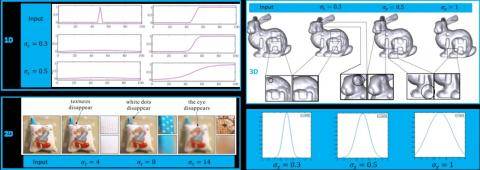Figure 1. The bilateral filtering results in 1D, 2D and 3D cases at different scale parameters σs

## 2.jpg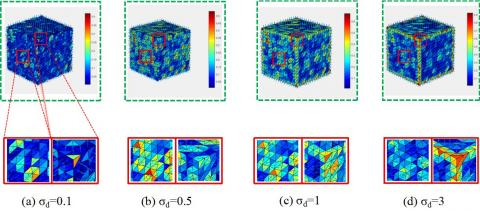Figure 2. The proposed scale descriptors at different scale parameters σd

4.1 Construction of scale descriptor

The previous section shows that the convolution kernel of the bilateral filter on 3D model consists of Gaussian functions based on face normals and spatial positions. In essence, bilateral filtering is a local averaging process in the neighborhood of the target vertex. If the scale parameter not configured properly, there will be severe loss of feature information. Traditionally, bilateral filtering adopts a fixed scale parameter, that is, the face normal of the entire 3D model are filtered with the same scale parameter. However, the model features on different scales should be treated differently based on their locations.

Therefore, this paper designs a way to configure the scale parameter adaptively. The scale descriptor was defined as:

$D({{n}_{i}})={{\sum\limits_{i}{{{A}_{i}}||{{n}_{i}}-{{K}_{i}}\sum\limits_{j\in N(i)}{{{\omega }_{ij}}{{n}_{j}}}||}}^{2}}$   (5)

where, Ai is the area of triangular face i; $K_{i}=\frac{1}{\sum_{j \in N(i)} \omega_{i j}}$ is the normalization operator; ωij=ωcωdAi (ωd is defined with the scale parameter σd); the Gaussian functions are as defined in the above section.

Figure 2 illustrates the proposed scale descriptors at different scale parameters σd. When the value of σd was small, the scale descriptor helped to highlight the noises on the surface of the cube model. With the gradual growth in σd, the scale descriptor facilitated the detection of features on larger scales. Since the scale descriptor can describe different scales of features, the noises were highlighted at a small σd (Figure 2(a)).

4.2 Local filter based on adaptive scale parameters

Based on the features of the scale descriptor, the scale parameter σs was configured adaptively for bilateral filtering, i.e. different σs values were set for different faces in the light of the scale descriptor of the target face.

As mentioned above, the scale descriptor helps to identify the noises of the model at a small σd. This means the scale descriptor increases with the level of noise. Thus, the scale parameter σs should be increased to realize harder smoothing at a high level of noise. Hence, the adaptive scale parameter was defined as:

$\overset{\tilde{\ }}{\mathop{{{\sigma }_{s}}}}\,=(\sigma _{s}^{1},\sigma _{s}^{2},\ldots ,\sigma _{s}^{M})$  (6)

where, si s can be expressed as:

$\sigma _{s}^{i}=\alpha *\exp (D({{n}_{i}}))$  (7)

where, α is an empirical parameter in (1, 2). The value of α is positively correlated with the noise level of the model.

The above formulas demonstrate that the scale parameter $\tilde{\sigma}_{s}$ is a vector, whose values correspond to the scale parameters of individual faces. In this way, the difference between faces could be considered in bilateral filtering. Moreover, the scale parameter was linked up with the scale descriptor D(ni), eliminating the need to manually adjust this sensitive parameter.

Based on the adaptive scale parameter, the bilateral filter can be modified as:

$n_{i}^{t+1}=\overset{\tilde{\ }}{\mathop{{{K}_{i}}}}\,\sum\limits_{j\in N(i)}{\overset{\tilde{\ }}{\mathop{{{\omega }_{ij}}}}\,n_{j}^{t}}$   (8)

where, $\tilde{\omega}_{i j}=\tilde{\omega}_{s} \tilde{\omega}_{c} A_{i}$; $\tilde{\omega}_{s}$ is the Gaussian function with element i of $\tilde{\sigma_{s}}$ as its scale parameter; $\tilde{K}_{i}$ is the normalization factor.

4.3 Termination criterion

To avoid the manual termination of the iterative process, an automatic termination was designed based on the minimization of the following objective function:

$J(t)=\sum\limits_{i=1}^{M}{D_{i}^{t}}+\beta \sum\limits_{i=1}^{M}{||\mathbf{L}(n_{i}^{t})|{{|}^{2}}}$  (9)

where, β is the balance parameter between the first term (sum of differences) and the second term (smooth measurement); L is the Laplacian operator on face normals, similar to the operator on vertices  with bilateral weights.

## 3.jpgFigure 3. The effectiveness of the objective function in measuring the denoising results

Figure 3(a) shows the convergence of our iterative scheme measured by mean squared error (MSE) and Figure 3(b) shows the variation of the objective function with the number of iterations. Under different values of β, the objective function was minimized in four or five iterations, showing visually ideal denoising results. The results show that the objective function is effective in measuring the denoising results.

After the face normals are filtered, Sun et al.  method was employed to update the vertex positions according to the face centroids and face normals:

$\overset{\tilde{\ }}{\mathop{{{v}_{i}}}}\,={{v}_{i}}+\frac{1}{|{{P}_{v}}(i)|}\sum\limits_{j\in {{P}_{v}}(i)}{n_{i}^{t+1}(n_{j}^{t+1}({{c}_{j}}-{{v}_{j}}))}$  (10)

where, vi and $\tilde{v}_{i}$ are the original and updated vertex positions, respectively; Pv(i) is the neighbors of vertex i; |Pv(i)| is the number of the neighbors of vertex i.

Then, the algorithm of our adaptive local filter for bilateral filtering was detailed in Algorithm 1.

 Algorithm 1: Adaptive local filter for bilateral filtering Input: A noisy model Output: A denoised model Step 1. Find the face normal {ni} of the noisy model. Step 2. Pre-filter the noisy model by any denoising method to preliminarily estimate the face normals $n_{0}^{i}$. Step 3. for t= 0 → ts do (ts is set based on the termination criterion.) (1) Calculate the scale descriptor by formula (5) based on $n_{i}^{t}$ (with a fixed σd); (2) Calculate the adaptive scale parameter by formula (6); (3) Update the face normals by formula (8) end for Step 4. Update the vertex positions by formula (10) based on the final $n_{i}^{t}$.

Global methods are generally more stable than local methods, thanks to their non-iterative nature. Zheng et al.  designed a well-known global method based on the following global optimization model:

$\overset{\tilde{\ }}{\mathop{{{n}_{i}}}}\,=\underset{\overset{\tilde{\ }}{\mathop{{{n}_{i}}}}\,}{\mathop{\arg \min }}\,\ (1-\lambda )\sum\limits_{i=1}^{M}{{{A}_{i}}\mathbf{L}(\overset{\tilde{\ }}{\mathop{{{n}_{i}}}}\,)}+\lambda \sum\limits_{i=1}^{M}{{{A}_{i}}||\overset{\tilde{\ }}{\mathop{{{n}_{i}}}}\,-{{n}_{i}}||_{2}^{2}}$  (11)

where, Ai is the area of face i. It is widely agreed that geometric details (e.g. high-frequency information like noises, tiny features, and weak features) can be smoothed effectively by minimizing the Laplacian operator. The minimization of the Laplacian operator can smooth the model surfaces without considering the features and noises. Thus, the loss of features depends on the scale parameter in the Laplacian operator.

Zheng et al.  configured the Laplacian operator non-homogenously, but the faces of the model are smoothed in the same intensity. However, the normals on the noisy areas should be smoothed more intense than those in feature areas, owing to the regional difference in feature scale. To solve the problem, our global method was designed as follows:

$\overset{\tilde{\ }}{\mathop{{{n}_{i}}}}\,=\underset{\overset{\tilde{\ }}{\mathop{{{n}_{i}}}}\,}{\mathop{\arg \min }}\,\ (1-\lambda )\sum\limits_{i=1}^{M}{{{A}_{i}}\overset{{}}{\mathop{\mathbf{\tilde{L}}(\overset{\tilde{\ }}{\mathop{{{n}_{i}}}}\,)}}\,}+\lambda \sum\limits_{i=1}^{M}{{{A}_{i}}||\overset{\tilde{\ }}{\mathop{{{n}_{i}}}}\,-{{n}_{i}}||_{2}^{2}}$  (12)

where, $\tilde{L}(\tilde{n_{i}})$ can be defined as:

$\mathbf{\tilde{L}}(\overset{\tilde{\ }}{\mathop{{{n}_{i}}}}\,)=\sum\limits_{i}{{{A}_{i}}||{{n}_{i}}-\overset{\tilde{\ }}{\mathop{{{K}_{i}}}}\,\sum\limits_{j\in N(i)}{\overset{\tilde{\ }}{\mathop{{{\omega }_{ij}}{{n}_{j}}|{{|}^{2}}}}\,}}$  (13)

where, $\tilde{\omega}_{i j}=\omega_{c} \tilde{\omega}_{s} A_{i}$. Similar to the local filter, the scale parameter $\tilde{\sigma}_{s}$ was calculated based on the scale descriptor. The scale parameter is still a vector, whose values correspond to the scale parameters of individual faces. Note that the scale descriptor in the global method was computed solely based on the model prefiltered by any simple method. This helps to estimate the general structure of the noisy model.

6. Experiments and Results Analysis

To verify its effectiveness, our filters were applied to experiments on real-world and synthetic models. Some models have holes (e.g. bunny) and some have different sampling rates (e.g. pyramid). The models were added different levels of noises: 0.5 Gaussian noise to the pyramid model; 0.1, 0.3 and 0.5 Gaussian noises to the cube model; 0.3 Gaussian noise to fandisk, bunny, skull, and angel models, respectively. The synthetic noise was generated from a zero-mean Gaussian with standard deviations proportional to the main edge length of the mesh.

All experiments were conducted on a computer (Intel® Core™ i7-7500U Processor (3.5GHz); 16GB memory). The pseudocode was implemented on the MATLAB. The runtimes of our filters and other local and global filters on the models are compared in Table 1. The comparison shows that our local and global filters are both efficient.

Table 1. The runtimes of different local and global filters

 Models (#V, #F) Time (s) Zheng  (Local) Zhang  Our filter (Local) Zheng  (Global) He  Our filter (Global) Cube (1538,3072) 0.354 0.631 0.457 0.308 0.825 0.386 Fandisk (6475,12946) 0.578 0.836 0.315 1.235 3.330 1.319 Bunny (34834,69451) 2.904 4.194 1.469 11.452 22.653 12.195
6.1 Properties of our filters

6.1.1 Robustness to different noise levels

To verify its robustness to heavy noises, our filters were adopted to denoise the cube models added 0.1, 0.3 and 0.5 Gaussian noises, respectively. The original model is shown in Figure 4(a); the three noisy models are presented in Figures 4(b)-(d), respectively; the results of our local filter and our global filter are displayed in the green box and red box of Figure 4, respectively.

As shown in Figure 4, both our filters removed the noises and preserved the model features, even if the model was contaminated with heavy noises. The good performance is attributable to the adoption of the adaptive scale parameters, which help to recover the geometric details in both local and global algorithms.

6.1.2 Robustness to irregular sampling

Next, our filters were adopted to denoise the pyramid models with different sampling rates. The original models and filtered results are shown in the upper row Figure 5. The experiment shows that both filters output visually correct results at different sampling rates. Our local filter did better in preserving geometric features, and obtained uniform filtering results. The excellence of our filters comes from the consideration of the area of faces.

6.1.3 Robustness to defects

In addition, our filters were employed to denoise the bunny models contaminated with different types of defects, including holes and open boundaries. The original models and filtered results are shown in the lower row Figure 5. It is learned that our filters smoothened the defects and preserved all the boundaries, an evidence of their robustness to defects.

## 4.jpgFigure 4. The experiments on the robustness to different noise levels

## 5.jpg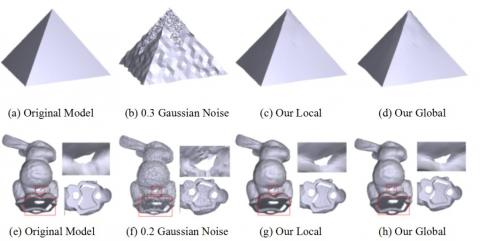Figure 5. The experiments on the robustness to irregular sampling and defects

6.2 Qualitative and quantitative comparisons

Our filters were compared with the state-of-the-arts. For fairness, the parameters of each method were finetuned carefully. The contrastive methods and their parameters are as follows:

The bilateral filter proposed by Fleishman et al.  (n1), the robust statistics proposed by Jones et al.  (σr, σg), the unilateral filtering algorithm proposed by Sun et al.  (T, n1, n2), the normal filter proposed by Zhang et al.  (r, σr, Kiter, Viter), the L0 minimization proposed by He and Schaefer  (λ1, α0, β0, uα, βmax), the global (λ2, σs1, n1) and local (σs2, n1, n2) filters proposed by Zheng et al. , and the robust feature-preserving denoising method proposed by Yadav et al.  (σs, σc, Viter).

Among the above methods, He and Yadav’s strategies are global methods, while the other strategies are local methods. Our local and global filters each contain four parameters: σd, n1, n2 and α1 for our local filter, and σd, λ, n2 and α for our global filter. Specifically, σd is the scale parameter depending on the noise level of the mesh model; n1 is the number of iterations to update the normal; n2 is the number of iterations to update vertex positions; λ is the balance parameter for our global filter; α is the scale factor. In our experiments, σd was empirically set to the range of [0.2, 1]; the greater the value of σd, the higher the noise level.

6.2.1 Quantitative comparisons

The parameters of the above methods were configured as shown in Table 2.

Table 2. The filtering results of multiple methods

 Models Methods Parameter settings MSAEs Fleishman  (12) 0.29323 Jones  (1.2,1.4) 0.26490 Sun  (0.35,5,20) 0.09926 Zheng  Local (0.35,50,20) 0.17793 Pyramid Zheng  Global (0.001,0.5,20) 0.16299 V=3455 He  (0.001,0.002,0.001,0.5,1.414,1000) 0.03773 Zhang  (2,0.45,50,20) 0.09872 Yadav  (0.55,0.2,100) 0.16425 Our Local (2,100,20,2) 0.08129 Our Global (0.6,0.01,20,2) 0.06183 Fleishman  (12) 0.16431 Jones  (1.8,1.5) 0.17749 Sun  (0.55,20,40) 0.03542 Zheng  Local (0.45,12,30) 0.05133 Cube Zheng  Global (0.005,0.5,20) 0.09198 V=1538 He  (0.01,0.00346,0.001,0.5,1.414,1000) 0.08169 Zhang  (2,0.25,25,20) 0.03077 Yadav  (0.55,0.2,100) 0.05133 Our Local (0.4,20,20,1.5) 0.01535 Our Global (0.4,0.01,20,1) 0.01560 Fleishman  (10) 0.12111 Jones  (1.3,1.4) 0.16946 Sun  (0.55,20,40) 0.07141 Zheng  Local (0.4,15,20) 0.06150 Fandisk Zheng  Global (0.35,0.0001,20) 0.11638 V=6475 He  (0.01,0.00346,0.001,0.5,1.414,1000) 0.09986 Zhang  (2,0.25,25,20) 0.05201 Yadav  (0.35,0.2,100) 0.06591 Our Local (0.4,10,20,2) 0.06048 Our Global (0.35,0.01,20,1) 0.05875 Fleishman  (12) 0.12094 Jones  (1.2,1.6) 0.10144 Sun  (0.5,6,20) 0.10279 Zheng  Local (0.5,6,10) 0.14032 Skull Zheng  Global (0.5,0.01,10) 0.14491 V=20002 He  (0.000004,1,0.001,0.9,1.414,1000) 0.12591 Zhang  (0.35,0.2,100) 0.09384 Yadav  (0.55,0.2) 0.12467 Our Local (0.3,3,20,1.5) 0.15255 Our Global (0.5,0.01,20,1) 0.15655 Fleishman  (5) 0.17071 Jones  (1.2,1.5) 0.18260 Sun  (0.5,5,20) 0.17818 Zheng  Local (0.5,4,20) 0.09209 iH-bunny Zheng  Global (0.5,0.01,20) 0.09723 V=34834 He  (0.000004,1,0.001,0.5,1.414,1000) 0.18032 Zhang  (2.7,0.55,4,15) 0.19706 Yadav  (0.55,0.2) 0.20332 Our Local (0.3,2,20,1) 0.09435 Our Global (0.3,0.05,20,1) 0.09300

The filtering results of each method were evaluated by mean square angular error (MSAE):

$MSAE=\mathbf{E}[(n_{i}^{GT},{{\tilde{n}}_{i}})]$   (14)

where, $n_{i}^{G T}$ and $\tilde{n}_{i}$ are the ground truth and filtered face normals, respectively; E[×] is the expectation. Table 2 shows that our local and global filters both obtained low MSAEs on different models.

6.2.2 Qualitative comparisons

Our filters were compared with the state-of-the-arts on two kinds of models: real-world models and synthetic models.

(1) Synthetic models

The synthetic models include fandisk, skull and bunny. Each model was added different levels of Gaussian noises.

The fandisk model (Figure 6) contains both strong and weak features, which are the focal points of denoising algorithms. The features on the models filtered by different methods were detected by the same method, i.e. the method proposed by Wang et al. . Hence, the detection accuracy is positively related with the effectiveness of feature-preserving denoising. It can be seen that our local and global filters preserved the strong and weak features well, laying the basis for restoration of the original model.

The filtering results on skull model and bunny model (Figures 7 and 8) demonstrate that our filters output better feature-preserving results than the other methods, as evidenced by the zoom-in subgraphs. The other methods removed the curve and teeth of the skull model to different degrees, although all of them could remove the noises. By contrast, our filters discriminated between features and noises, thus preserved the geometric details well.

Our filters were compared with the said methods on the pyramid model with irregular sampling rates and the cube model with 0.5 Gaussian noise. The results in Figures 9 and 10 show that, whether it is cube model or pyramid model, our filters outperformed the other methods.

According to the MSAEs in Table 2, our local and global filters had much smaller MSAEs than the other methods, regardless of cube model, pyramid model, and fandisk model. On complex models like bunny and skull, our MSAEs were smaller than or equal to those of other methods. To sum up, both visual analysis and numerical analysis confirm the robustness of our filters.

## 6.jpg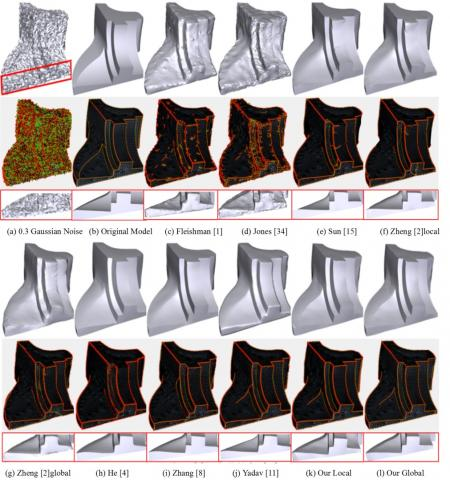Figure 6. The denoising effects of different methods on fandisk model

## 7.jpg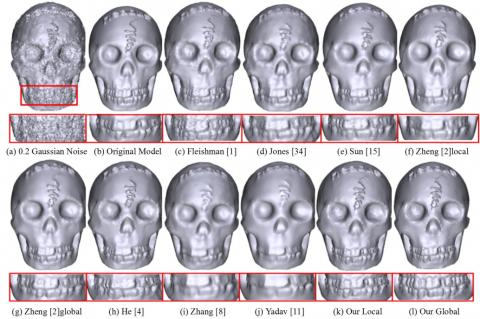Figure 7. The denoising effects of different methods on skull model

## 8.jpg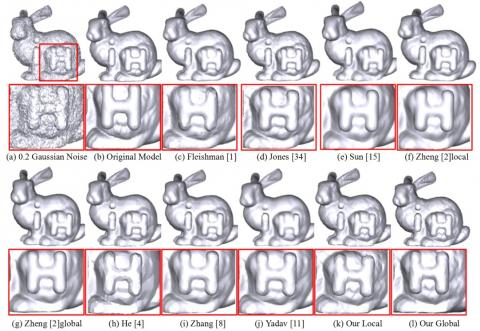Figure 8. The denoising effects of different methods on bunny model

## 9.jpg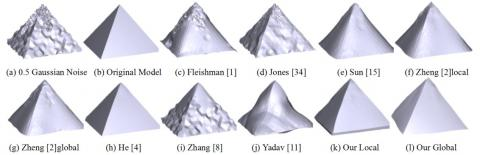Figure 9. The denoising effects of different methods on pyramid model

## 10.jpg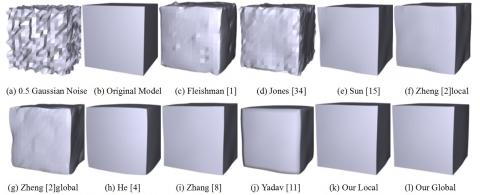Figure 10. The denoising effects of different methods on cube model

(2) Real-world models

Furthermore, our filters were compared with other methods on real-world models, namely, angel, rabbit, and Wilhelm. The original models contain little noises. Before the experiments, each model was added different kinds of defects, such as open boundaries and holes.

The filtering results on angel, rabbit, and Wilhelm models are displayed in Figures 11-13, respectively. It can be seen that our filters removed the noises and preserved the geometric details, especially the eyes, better than the contrastive methods in the angel model; our filters maintained the muscle features of the rabbit model more accurately than the other methods; our filters also preserved the eyes and hair of the Wilhelm model better than the other methods. In summary, our filters achieved desirable effects in noise removal and feature preservation.

## 11.jpg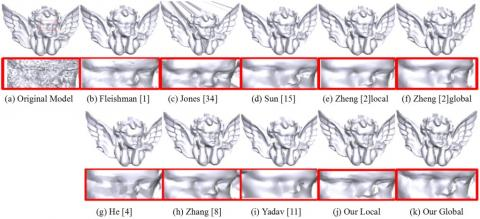Figure 11. The denoising effects of different methods on angel model

## 12.jpg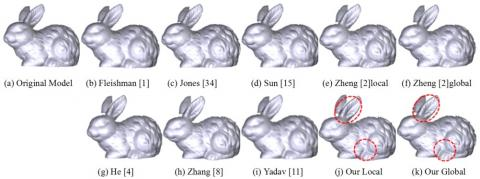Figure 12. The denoising effects of different methods on rabbit model

## 13.jpg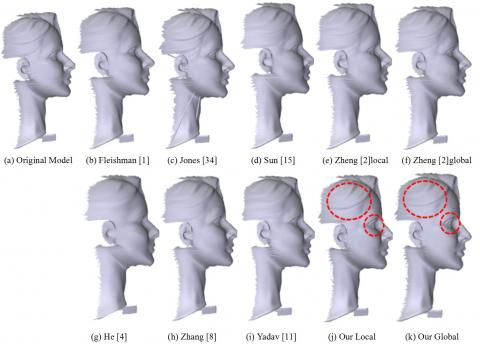Figure 13. The denoising effects of different methods on Wilhelm model

7. Conclusions

This paper introduces the adaptive scale parameters to modify the classical bilateral filtering method. The adaptive scale parameters were constructed based on the analysis on Gaussian scale space. Besides, the authors defined feature scales and scale descriptors on 3D models, bridging the gap between scale space analysis and feature scales on these models. On this basis, adaptive 3D local and global filters were designed, which configure the scale parameter for each face normal in an adaptive manner. Quantitative and qualitative analyses show that our filters are equivalent to or superior than the state-of-the-arts in noise removal and feature preservation.

Acknowledgment

This work was supported by the National Natural Science Foundation of China (Grant No.: 61802045), and the Open Project Program of State Key Laboratory of Virtual Reality Technology and Systems, Beihang University (Grant No.: VRLAB2020A04).

References

 Fleishman, S., Drori, I., Cohen-Or, D. (2003). Bilateral mesh denoising. ACM Transactions on Graphics, 22(3): 950-953. https://doi.org/10.1145/1201775.882368

 Zheng, Y., Fu, H., Au, O.K.C., Tai, C.L. (2010). Bilateral normal filtering for mesh denoising. IEEE Transactions on Visualization and Computer Graphics, 17(10): 1521-1530. https://doi.org/ 10.1109/TVCG.2010.264

 Centin, M., Signoroni, A. (2018). Mesh denoising with (Geo) metric fidelity. IEEE Transactions on Visualization and Computer Graphics, 24(8): 2380-2396. https://doi.org/10.1109/tvcg.2017.2731771

 He, L., Schaefer, S. (2013). Mesh denoising via L0 minimization. ACM Transactions on Graphics, 32(4): 1-8. https://doi.org/ 10.1145/2461912.2461965

 Liu, Z., Zhong, S., Xie, Z., Wang, W. (2019). A novel anisotropic second order regularization for mesh denoising. Computer Aided Geometric Design, 71: 190-201. https://doi.org/10.1016/j.cagd.2019.04.013

 Wang, P.S., Liu, Y., Tong, X. (2016). Mesh denoising via cascaded normal regression. ACM Transactions on Graphics, 35(6): 1-12. https://doi.org/10.1145/2980179.2980232

 Wang, J., Huang, J., Wang, F.L., Wei, M., Xie, H., Qin, J. (2019). Data-driven geometry-recovering mesh denoising. Computer-Aided Design, 114: 133-142. https://doi.org/10.1145/2980179.2980232

 Zhang, W., Deng, B., Zhang, J., Bouaziz, S., Liu, L. (2015). Guided mesh normal filtering. Computer Graphics Forum, 34(7): 23-34. https://doi.org/10.1111/cgf.12742

 Liu, L., Peng, F. (2009). Adaptive algorithm of scale parameter based on scale-space. Proceedings of SPIE - the International Society for Optical Engineering, 7496: 74962K. https://doi.org/10.1117/12.833665

 Liu, B., Cao, J., Wang, W., Ma, N., Li, B., Liu, L., Liu, X. (2018). Propagated mesh normal filtering. Computers & Graphics, 74: 119-125. https://doi.org/10.1016/j.cag.2018.05.003

 Yadav, S.K., Reitebuch, U., Polthier, K. (2019). Robust and high fidelity mesh denoising. IEEE Transactions on Visualization and Computer Graphics, 25(6): 2304-2310. https://doi.org/10.1109/tvcg.2018.2828818

 Wang, P.S., Fuz, X.M., Liu, Y., Tong, X., Liu, S.L., Guo, B. (2015). Rolling guidance normal filter for geometric processing. ACM Transactions on Graphics, 34(6cd): 173.1-173.9. https://doi.org/10.1145/2816795.2818068

 Zheng, Y., Li, G., Xu, X., Wu, S., Nie, Y. (2018). Rolling normal filtering for point clouds. Computer Aided Geometric Design, 62: 16-28. https://doi.org/10.1016/j.cagd.2018.03.004

 Liu, S., Rho, S., Wang, R., Jiang, F. (2018). Feature-preserving mesh denoising based on guided normal filtering. Multimedia Tools and Applications, 77(2): 1-13. https://doi.org/ 10.1007/s11042-018-5735-9

 Sun, X., Rosin, P.L., Martin, R.R., Langbein, F.C. (2007). Fast and effective feature-preserving mesh denoising. IEEE Transactions on Visualization and Computer Graphics, 13(5): 925-938. https://doi.org/10.1109/TVCG.2007.1065

 Li, T., Wang, J., Liu, H., Liu, L.G. (2017). Efficient mesh denoising via robust normal filtering and alternate vertex updating. Frontiers of Information Technology & Electronic Engineering, 18(11): 1828-1842. https://doi.org/10.1631/FITEE.1601229

 Hurtado, J., Gattass, M., Raposo, A., Coelho, J. (2018). Adaptive patches for mesh denoising. Brazilian Symposium on Computer Graphics and Image, https://doi.org/10.1109/SIBGRAPI.2018.00007

 Zhang, H., Wu, C., Zhang, J., Deng, J. (2015). Variational mesh denoising using total variation and piecewise constant function space. IEEE Transactions on Visualization and Computer Graphics, 21(7): 873-886. https://doi.org/10.1109/tvcg.2015.2398432

 Zhao, Y., Qin, H., Zeng, X., Xu, J., Dong, J. (2018). Robust and effective mesh denoising using L0 sparse regularization. Computer-Aided Design, 101: 82-97. https://doi.org/10.1016/j.cad.2018.04.001

 Wang, R., Yang, Z., Liu, L., Deng, J., Chen, F. (2014). Decoupling noise and features via weighted L1-analysis compressed sensing. ACM Transactions on Graphics, 33: 2. https://doi.org/10.1145/2557449

 Zhong, S., Xie, Z., Liu, J., Liu, Z. (2019). Robust Mesh Denoising via Triple Sparsity. Sensors, 19(5): 1101. https://doi.org/10.3390/s19051001

 Wei, M., Liang, L., Pang, W., Wang, J., Li, W., Wu, H. (2017). Tensor Voting Guided Mesh Denoising. IEEE Transactions on Automation Science and Engineering, 14(2): 931-945. https://doi.org/10.1109/TASE.2016.2553449

 Yadav, S.K., Reitebuch, U., Polthier, K. (2018). Mesh Denoising Based on Normal Voting Tensor and Binary Optimization. IEEE Transactions on Visualization and Computer Graphics, 24(8): 2366-2379. https://doi.org/10.1109/TVCG.2017.2740384

 Xing, Y., Bai, L., Tan J., Hong, P. (2018). Robust mesh denoising based on collaborative filters. Journal of Advanced Mechanical Design Systems and Manufacturin, 12(4): JAMDSM0084. https://doi.org/10.1299/jamdsm.2018jamdsm0084

 Lu, X., Chen, W., Schaefer, S. (2017). Robust mesh denoising via vertex pre-filtering and l1-median normal filtering. Computer Aided Geometric Design, 54: 49-60. https://doi.org/10.1016/j.cagd.2017.02.011

 Li, T., Liu, W., Liu, H., Wang, J., Liu, L. (2019). Feature-convinced mesh denoising. Graphical Models, 101: 17-26. https://doi.org/10.1016/j.gmod.2018.12.002

 Wei, M., Yu, J., Pang, W.M., Wang, J., Qin, J., Liu, L., Heng, P.A. (2014). Bi-normal filtering for mesh denoising. IEEE Transactions on Visualization and Computer Graphics, 21(1): 43-55. https://doi.org/10.1109/TVCG.2014.2326872

 Wei, M., Huang, J., Xie, X., Liu, L., Wang, J., Qin, J. (2018). Mesh denoising guided by patch normal co-filtering via kernel low-rank recovery. IEEE Transactions on Visualization and Computer Graphics, 25(10): 2910-2926. https://doi.org/10.1109/TVCG.2018.2865363

 Wang, J., Zhang, X., Yu, Z. (2012). A cascaded approach for feature-preserving surface mesh denoising. Computer-Aided Design, 44(7): 597-610. https://doi.org/10.1016/j.cad.2012.03.001

 Lu, X., Deng, Z., Chen, W. (2015). A robust scheme for feature-preserving mesh denoising. IEEE Transactions on Visualization and Computer Graphics, 22(3): 1181-1194. https://doi.org/10.1109/TVCG.2015.2500222

 Tomasi, C., Manduchi, R. (1998). Bilateral filtering for gray and color images. In Sixth international conference on computer vision. IEEE Cat. No. 98CH36271: 839-846. https://doi.org/10.1109/ICCV.1998.710815

 Nealen, A., Igarashi, T., Sorkine, O., Alexa, M. (2006, November). Laplacian mesh optimization. In Proceedings of the 4th international conference on Computer graphics and interactive techniques in Australasia and Southeast Asia, 381-389. https://doi.org/10.1145/1174429.1174494

 Sun, X., Rosin, P.L., Martin, R., Langbein, F. (2007). Fast and effective feature-preserving mesh denoising. IEEE Transactions on Visualization and Computer Graphics, 13(5): 925-938. https://doi.org/10.1109/TVCG.2007.1065

 Jones, T.R., Durand, F., Desbrun, M. (2003). Non-iterative, feature-preserving mesh smoothing. In ACM SIGGRAPH 2003, 943-949.

 Wang, X.C., Cao, J.J., Liu, X.P., Li, B.J., Shi, X.Q., Sun, Y.Z. (2012). Feature detection of triangular meshes via neighbor supporting. Journal of Zhejiang University Science C, 13(6): 440-451.